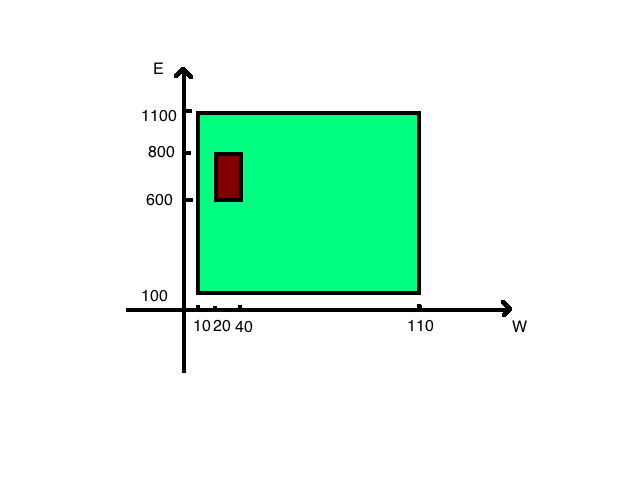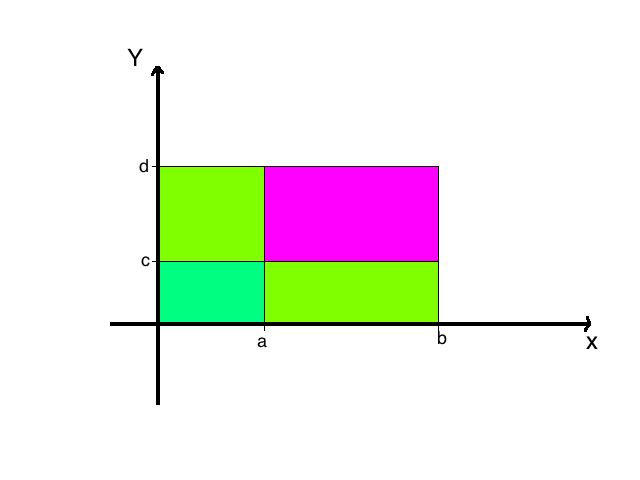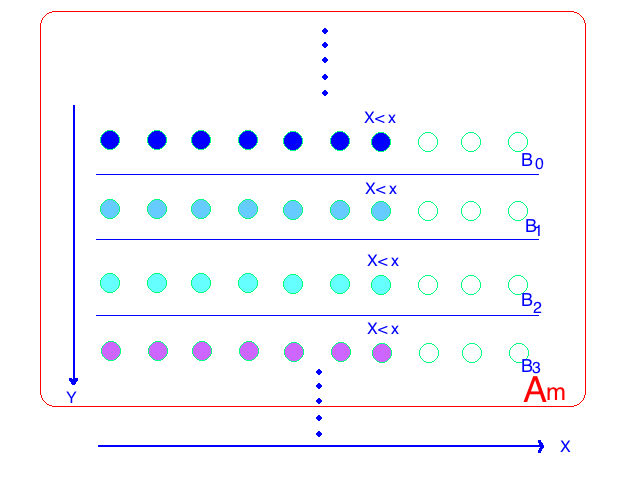Abstract: 本文主要介绍双变量的分布情况，以及其中的一些有用的性质
Keywords: Discrete Joint Distribution,Continuous Joint Distribution,Mixed Bivariate Distribution,Bivariate Cumulative Distribution Functions

# 二维分布

## 联合分布 Joint Distribution

$$\text{Bivariate}= \begin{cases} Discrete & \text{Discrete,Discrete}\\ Continuous & \text{Continuous,Continuous}\\ Hybrid & \text{Discrete,Continuous} \end{cases}$$

Definition Joint/Bivariate Distribution:Let $X$ and $Y$ be random varibales.The joint distribution or bivariate distribution of $X$ and $Y$ is the collection of all probabilities of the form $Pr[(X,Y)\in C]$ for all sets $C$ of pairs of real numbers such that ${(X,Y)\in C}$ is an event

## 离散联合分布 Discrete Joint Distribution

Definition Joint Distribution:Let X and Y be random variables,and consider the ordered pair(X,Y).If there are only finitely or at most countably many different possible values (x,y) for the pair (X,Y),then we say that x and Y have a discrete joint distribution

1 $\frac{1}{12}$ $\frac{1}{12}$
2 $\frac{1}{12}$ $\frac{1}{12}$
3 $\frac{1}{12}$ $\frac{1}{12}$
4 $\frac{1}{12}$ $\frac{1}{12}$
5 $\frac{1}{12}$ $\frac{1}{12}$
6 $\frac{1}{12}$ $\frac{1}{12}$

Theorem: Suppose that two random variable X and Y each have a discrete distribution.Then X and Y have a discrete joint distribution

1. 如果两个样本空间有限，那么其笛卡尔积有限，这个是集合论中已经明确的结论了
2. 如果两个样本空间可数无限，那么气笛卡尔积也是可数无限的，这个相关证明也在集合论中，这里不再证明

Definition Joint Probability Function,p.f. The joint probability function,or the joint p.f. of X and Y is defined as the function f such that for every point (x,y) in xy-plane:
$$f(x,y)=Pr(X=x\text{ and } Y=y)$$

Theorem Let $X$ and $Y$ have a discrete joint distribution.If $(x,y)$ is not one of the possible values of the pair $(X,Y)$ the $f(x,y)=0$ .Also,
$$\sum_{\text{All }(x,y)}f(x,y)$$
Finally,for each set $C$ of ordered pairs
$$Pr[(X,Y)\in C]=\sum_{(x,y)\in C}f(x,y)$$

$$Pr[(X,Y)\in C]=\frac{1}{12}+\frac{1}{12}+\frac{1}{12}=\frac{1}{4}$$

## 连续联合分布 Continuous Joint Distribution

$$Pr[(X,Y)\in C]=\int_C\int \frac{1}{100000}dx dy$$Definition Continuous Joint Distribution/Joint p.d.f./Support:Two random varibales X and Y have a continuous joint distribution if there exists a nonnegative function f defined over the entire xy-plane such that for every subset C of the plane,
$$Pr[(X,Y)\in C]=\int_C\int f(x,y)dx dy$$
if the integral exists.The function $f$ is called the joint probability density function(abbreviated joint p.d.f)of $X$ and $Y$.The closure of the set ${(x,y):f(x,y)>0}$ is called the support of (the distribution of) $(X,Y)$

A joint p.d.f. must satisfy the following two conditions:
$$f(x,y)\geq 0 \text{ for } -\infty <x<\infty \text{ and } -\infty <y<\infty$$
and
$$\int^{\infty}_{-\infty}\int^{\infty}_{-\infty}f(x,y)dxdy=1$$

Theorem For every contimuous joint distribution on the xy-plane,the following two statements hold:

1. Every individual point,and every infinite sequence of points,in the xy-plane has pribability 0.
2. Let $f$ be a continuous function of one real variable defined on a(possibly unbounded) interval(a,b).The sets ${(x,y):y=f(x),a<x<b}$ and ${(x,y):x=f(y),a<y<b}$ have probability 0

## 混合二维分布 Mixed Bivariate Distribution

Definition Joint p.f/p.d.f: Let $X$ and $Y$ be random variables such that $X$ is discrete and $Y$ is continuous.Suppose that there is a function $f(x,y)$ define on the xy-plane such that,for every pair A and B of subsets of the real numbers
$$Pr(X\in A \text{ and } Y \in B)=\int_B\sum_{x\in A}f(x,y)dy$$
if the integral exists.Then the function $f$ is called the joint p.f./p.d.f of $X$ and $Y$

$$\int^{\infty}_{-\infty}\sum^{\infty}_{i=1}f(x_i,y)dy=1$$

$$(X,Y)\in C\\ \text{we set } C_x={y:(x,y)\in C}\\ \text{then } Pr((X,Y)\in C)=\sum_{\text{All } x}\int_{C_x}f(x,y)dy$$

$$f(x,p)=p^x(1-p)^{1-x}\text{ for } x=0,1 \text{ and } 0<p<1$$

$$Pr(X\leq 0 \text{ and } P\leq \frac{1}{2})=\int_0^{\frac{1}{2}}(1-p)dp\\ =-\frac{1}{2}[(1-\frac{1}{2})^2-(1-0)^2]=\frac{3}{8}$$

## 二维累积分布函数 Bivariate Cumulative Distribution Functions

Definition: Joint(Cumulative) Distribution Function/c.d.f. The joint distribution function or joint cumulative distribution function (joint c.d.f) of two random variables X and Y is defined as the function F such that for all values of x and y( $-\infty<x<\infty$ and $-\infty<y<\infty$)
$$F(x,y)=Pr(X\leq x \text{ and } Y\leq y )$$

$$Pr(a<x\leq b \text{ and } c<Y\leq d)\\ =Pr(a<X\leq b \text{ and } y\leq d) -Pr(a<x\leq b \text{ and } y\leq c)\\ =[Pr(X\leq b \text{ and } Y \leq d)-Pr(X\leq a \text{ and } Y \leq d)]-\\ [Pr(X\leq b \text{ and } Y \leq c)-Pr(X\leq a \text{ and } Y \leq c)]\\ =F(b,d)-F(a,d)-F(b,c)+F(a,c)$$Theorem Let $X$ and $Y$ have a joint c.d.f. $F$.The c.d.f. $F_1$ of just the single random variable $X$ can be derived from the joint c.d.f. $F$ as $F_1(x)=lim_{y\to \infty}F(x,y)$.Similarly,the c.d.f. $F_2$ of $Y$ equals $F_2(y)=lim_{x\to \infty}F(x,y)$ ,for $0<y\leq \infty$1. 离散情况下：
$$\text{Let }\\ B_0={X\leq x \text{ and } Y\leq 0} \\ B_1={X\leq x \text{ and }n-1<y\leq n}\text{ ,for } n=1,2\dots\\ A_m=\bigcup^{m}_{n=0}B_n\text{ ,for }m=1,2,\dots\\$$
我们可以确定:${X\leq x}=\bigcup^{\infty}_{n=-0}B_n$ ，并且 $A_m={X\leq x \text{ and } Y\leq m} \text{ for } m=1,2,\dots$ 这样我们有 $Pr(A_m)=F(x,m) \text{ for each } m$

$$F_1(x)=Pr(X\leq x)=Pr(\bigcup^{\infty}_{n=1}B_n)\\ =\sum^{\infty}_{n=0}Pr(B_n)=lim_{m\to \infty} Pr(A_m)\\ =lim_{m\to \infty}F(x,m)=lim_{y\to \infty}Pr(A_m)$$

1. 连续情况下：
这个证明就简单多了和上述证明基本一致，只要把求和编程就积分就可以证明连续情况下的结论

$$F(x,y)=\int^y_{-\infty}\int^x_{-\infty}f(r,s)drds\\ f(x,y)=\frac{\partial^2F(x,y)}{\partial x\partial y}$$

0%